Tamilnadu State Board New Syllabus Samacheer Kalvi 8th Maths Guide Pdf Chapter 5 Geometry Ex 5.4 Text Book Back Questions and Answers, Notes.

## Tamilnadu Samacheer Kalvi 8th Maths Solutions Chapter 5 Geometry Ex 5.4

I. Construct the following quadrilaterals with the given measurements and also find their area.

Question 1.
ABCD, AB = 5 cm, BC = 4.5 cm, CD = 3.8 cm, DA = 4.4 cm and AC = 6.2 cm.
Given ABCD, AB = 5 cm, BC = 4.5 cm, CD = 3.8 cm, DA = 4.4 cm and AC = 6.2 cm.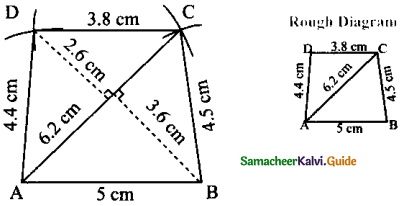Steps:

• Draw a line segment AB = 5 cm
• With A and B as centers drawn arcs of radii 6.2 cm and 4.5cm respectively and let them cut at C.
• Joined AC and BC.
• With A and C as centrers drawn arcs of radii 4.4cm and 3.8 cm respectively and let them at D.
• ABCD is the required quadrilateral.

Calculation of Area:
Area of the quadrilateral ABCD = $$\frac { 1 }{ 2 }$$ × d × (h11 + h2) sq. units
= $$\frac { 1 }{ 2 }$$ × 6.2 × (2.6 + 3.6)cm2 = 3.1 × 6.2 = 19.22 cm2Question 2.
PLAY, PL = 7cm, LA = 6cm, AY = 6cm, PA = 8cm and LY = 7cm.
Given PLAY, PL = 7cm, LA = 6cm, AY = 6cm, PA = 8cm and LY = 7cm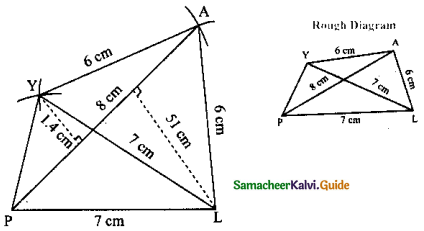Steps:

• Drawn a line segment PL = 7 cm
• With P and L as centers, drawn arcs of radii 8 cm and 6 cm respectively, let them cut at A.
• Joined PA and LA.
• With L and A as centers, drawn arcs of radii 7 cm and 6 cm respectively and let them cut at Y.
• Joined LY, PY and AY.
• PLAY is the required quadrilateral.

Calculation of Area:
Area of the quadrilateral PLAY = $$\frac { 1 }{ 2 }$$ × d × (h1 + h2) sq. units = $$\frac { 1 }{ 2 }$$ × 8 × (5.1 + 1.4) cm2
$$\frac { 1 }{ 2 }$$ × 8 × 6.5 cm2 = 26 cm2
Area of the quadrilateral = 26 cm2Question 3.
PQRS, PQ = QR = 3.5 cm, RS = 5.2 cm, SP = 5.3 cm and ∠Q = 120°.
Given PQ = QR = 3.5 cm, RS = 5.2 cm, SP = 5.3 cm and ∠Q = 120°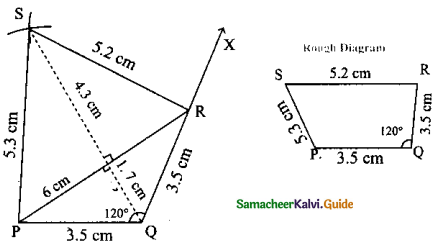Steps:

• Draw a line segment PQ = 3.5 cm
• Made ∠Q = 120°. Drawn the ray QX.
• With Q as centre drawn an arc of radius 3.5 cm. Let it cut the ray QX at R.
• With R and P as centres drawn arcs of radii 5.2cm and 5.5 cm respectively and let them cut at S.
• Joined PS and RS.
• PQRS is the required quadrilateral.

Calculation of Area:
Area of the quadrilateral PQRS = $$\frac { 1 }{ 2 }$$ × d × (h1 + h2) sq. units
= $$\frac { 1 }{ 2 }$$ × 6 × (4.3 + 17)cm2
= 3 × 6cm2
= 18 cm2
Area of the quadrilateral PQRS = 18 cm2Question 4.
MIND, MI = 3.6 cm, ND = 4 cm, MD = 4 cm, ∠M = 50° and ∠D = 100°.
Given MI = 3.6 cm, ND = 4 cm, MD = 4 cm, ∠M = 50° and ∠D = 100°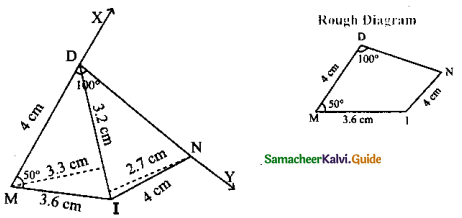Steps:

• Drawn a line segment MI = 3.6 cm
• At M on MI made an angle ∠IMX = 500
• Drawn an arc with center M and radius 4 cm let it cut MX it D
• At D on DM made an angle ∠MDY = 100°
• With I as center drawn an arc of radius 4 cm, let it cut DY at N.
• Joined DN and IN.
• MIND is the required quadrilateral.

Calculation of Area:
Area of the quadrilateral MIND = $$\frac { 1 }{ 2 }$$ × d × (h1 + h2) sq. units = $$\frac { 1 }{ 2 }$$ × 3.2 × (2.7 + 33) cm2
= $$\frac { 1 }{ 2 }$$ × 3.2 × 6 cm2 = 9.6 cm2
Area of the quadrilateral = 9.6 cm2Question 5.
AGRI, AG = 4.5 cm, GR = 3.8 cm, ∠A = 60°, ∠G = 110° and ∠R = 90°.
AG = 4.5 cm, GR = 3.8 cm, ∠A = 60°, ∠G = 110° and ∠R = 90°.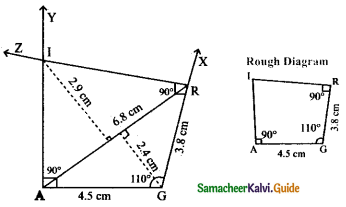Steps:

• Draw a line segment AG = 4.5 cm
• At G on AG made ∠AGX = 110°
• With G as centre drawn an arc of radius 3.8 cm let it cut GX at R.
• At R on GR made ∠GRZ = 90°
• At A on AG made ∠GAY = 90°
• AY and RZ meet at I.
• AGRI is the required quadrilateral.

Calculation of Area:
Area of the quadrilateral AGRI = $$\frac { 1 }{ 2 }$$ × d × (h1 + h2) sq. units = × 6.8 × (2.9 + 2.4) cm2
= $$\frac { 1 }{ 2 }$$ × 6.8 × 5.3 × cm2
Area of the quadrilateral = 18.02 cm2II. Construct the following trapeziums with the given measures and also find their area.

Question 1.
AIMS with $$\overline{\mathrm{AI}}$$ || $$\overline{\mathrm{SM}}$$, AI = 6cm, IM = 5cm, AM = 9cm and MS = 6.5cm.
Given AI = 6 cm, IM = 5 cm
AM = 9 cm, and $$\overline{\mathrm{AI}}$$ || $$\overline{\mathrm{SM}}$$
MS = 6.5 cm

Rough Diagram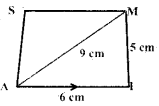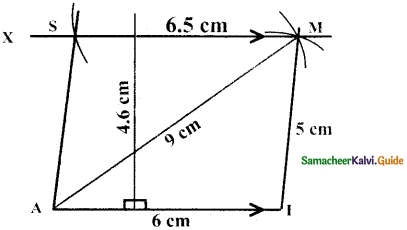Construction:
Steps:

• Draw a line segment AI = 6cm.
• With A and I as centres, draw arcs of radii 9 cm and 5 cm respectively and let them cut at M
• Join AM and IM.
• Draw MX parallel to AI
• With M as centre, draw an arc of radius 6.5 cm cutting MX at S.
• Join AS AIMS is the required trapezium.

Calculation of Area
Area of the trapezium AIMS = $$\frac { 1 }{ 2 }$$ × h × (a + b) sq.units
= $$\frac { 1 }{ 2 }$$ × 4.6 × (6 + 6.5)
= $$\frac { 1 }{ 2 }$$ × 4.6 × 12.5
= 28.75 Sq.cmQuestion 2.
CUTE with $$\overline{\mathrm{CD}}$$ || $$\overline{\mathrm{ET}}$$, CU = 7cm, ∠ UCE = 80° CE = 6cm and TE = 5cm.
Given: In the trapezium CUTE,
CU = 7cm, ∠UCE = 80°,
CE = 6cm,TE = 5cm and $$\overline{\mathrm{CD}}$$ || $$\overline{\mathrm{ET}}$$

Rough Diagram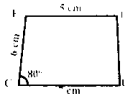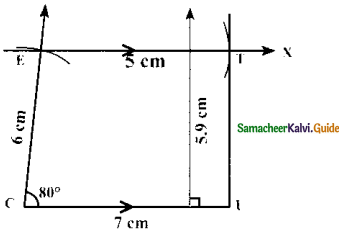Construction:
Steps:

• Draw a line segment CU = 7cm.
• Construct an angle ∠UCE = 80° at C
• With C as centre, draw an arc of radius 6 cm cutting CY at E
• Draw EX parallel to CU
• With E as centre, draw an arc 7 cm of radius 5 cm cutting EX at T
• Join UT. CUTE is the required trapezium.

Calculation of area:
Area of the trapezium CUTE = $$\frac { 1 }{ 2 }$$ × h × (a + b) sq. units
= $$\frac { 1 }{ 2 }$$ × 5.9 × (7 + 5)sq. units
= 35.4 sq.cmQuestion 3.
ARMY with $$\overline{\mathrm{AR}}$$ || $$\overline{\mathrm{YM}}$$, AR = 7cm, RM = 6.5 cm ∠RAY = 100° and ∠ARM = 60°
Given: In the trapezium ARMY
AR = 7cm,RM = 6.5cm,
∠RAY = 100° and ARM 60°, $$\overline{\mathrm{AR}}$$ || $$\overline{\mathrm{YM}}$$

Rough Diagram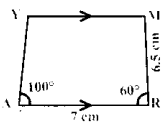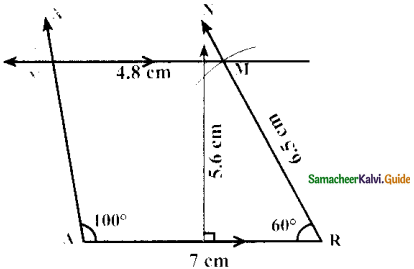Construction:
Steps:

• Draw a line segment AR = 7 cm.
• Construct an angle ∠RAX = 100° at A
• Construct an angle ∠ARN = 60° at R
• With R as centre, draw an arc of radius 6.5 cm cutting RN at M
• Draw MY parallel to AR
• ARMY is the required trapezium.

Calcualtion of Area:
Area of the trapezium ARMY = $$\frac { 1 }{ 2 }$$ × h × (a + b) sq. units
= $$\frac { 1 }{ 2 }$$ × 5.6 × (7 + 4.8) sq. units
= $$\frac { 1 }{ 2 }$$ × 5.6 × 1.18
= 33.04 sq.cmQuestion 4.
CITY with $$\overline{\mathrm{CI}}$$ || $$\overline{\mathrm{YT}}$$, CI = 7cm, IT = 5 .5 cm, TY = 4cm and YC = 6cm.
Given: In the trapezium CITY,
CI = 7cm,IT = 5.5cm, TY = 4cm
YC = 6cm, and $$\overline{\mathrm{CI}}$$ || $$\overline{\mathrm{YT}}$$

Rough Diagram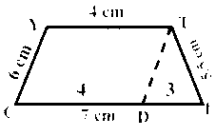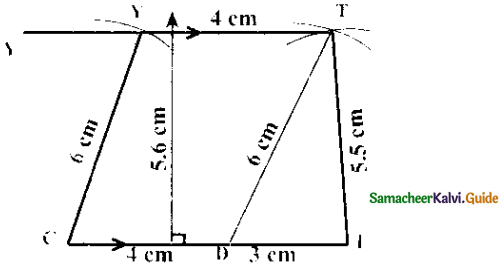Construction:
Steps:

• Draw a line segment CI = 7 cm.
• Mark a point D on CI such that CD = 4cm
• With D and I as centres, draw arcs of radii 6 cm and 5.5 cm respectively.
Let them cut at T. Join DT and IT.
• With C as centre, draw an arc of radius 6 cm.
• Draw TY parallel to Cl. Let the line cut the previous arc at Y.
• Join CY. CITY is the required trapezium.

Construction of area:
Area of the trapezium CITY = $$\frac { 1 }{ 2 }$$ × h × (a + b) sq. units
= $$\frac { 1 }{ 2 }$$ × 5.5 × (7 + 4)sq.units
= $$\frac { 1 }{ 2 }$$ × 5.5 × 11
= 30.25 sq.cm